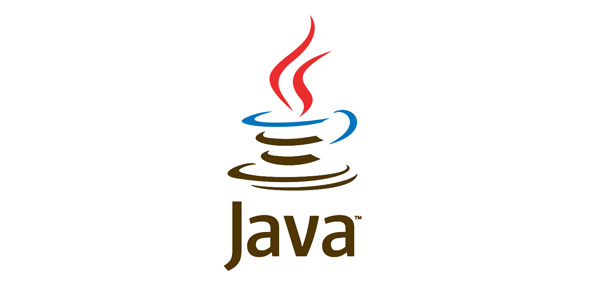# Java Programming Language Quiz Coding

20 Questions | Total Attempts: 1336SettingsTotal Questions=20

• 1.
--Controller Servlet in struts
• A.

ActionServlet

• B.

FrontControllerServlet

• C.

FormBean class

• D.

Action class

• 2.
Which of the collection classes will follow the InsertionOrder
• A.

ArrayList

• B.

• C.

Vector

• D.

All the above

• 3.
Http protocol is a _____________
• A.

State full

• B.

State less

• C.

Both

• D.

None

• 4.
Public static void parse(String str) {  try {  float f = Float.parseFloat(str);  } catch (NumberFormatException nfe) {  f = 0;  } finally {  System.out.println(f);  }  }  public static void main(String[] args) {  parse("invalid");  } What is the result?
• A.

0.0

• B.

Compilation fails.

• C.

A ParseException is thrown by the parse method at runtime

• D.

A NumberFormatException is thrown by the parse method at runtime.

• 5.
Public class Yippee2 {  static public void main(String [] yahoo) {  for(int x = 1; x < yahoo.length; x++) {  System.out.print(yahoo[x] + " ");  } . }  } and the command line invocation: java Yippee2 a b c What is the result?
• A.

A b

• B.

B c

• C.

a b c

• D.

Compilation fails.

• E.

An exception is thrown at runtime.

• 6.
Public class Test {  public static void main(String [] args) {  int x = 5; boolean b1 = true;  boolean b2 = false; f ((x == 4) && !b2 )  System.out.print("1 "); System.out.print("2 ");. if ((b2 = true) && b1 )  System.out.print("3 "); }  } What is the result?
• A.

2

• B.

3

• C.

1 2

• D.

2 3

• 7.
Public class Hello { String title;  int value; public Hello() {  title += " World";  }  public Hello(int value) {  this.value = value;  title = "Hello";  Hello();  }  } and:  Hello c = new Hello(5); System.out.println(c.title); What is the result?
• A.

Hello

• B.

Hello World

• C.

Compilation fails.

• D.

Hello World 5

• 8.
Public class Wow {  public static void go(short n) {System.out.println("short");}  public static void go(Short n) {System.out.println("SHORT");}  public static void go(Long n) {System.out.println(" LONG");}  public static void main(String [] args) {  Short y = 6;  int z = 7;  go(y);  go(z);  }  } What is the result?
• A.

Short LONG

• B.

SHORT LONG

• C.

Compilation fails.

• D.

An exception is thrown at runtime.

• 9.
Which of the following are correct as per MVC architecture
• A.

Two jsp files should interact with each other.

• B.

Every web application should contain only one controller.

• C.

View can directly interact with model.

• D.

A web application can contain 4 layers

• 10.
<% int a = 10; %> <%! int a = 20; %> <%! int b = 30; %> The value of b multiplied by a is <%= b * a %>
• A.

The code will not compile

• B.

The value of b multiplied by a is 30

• C.

The value of b multiplied by a is 300

• D.

The value of b multiplied by a is 600

• 11.
If i want to use the name of the person across multiple pages then we have to store that person name in ___
• A.

Request

• B.

Session

• C.

Application

• D.

Servletrequest

• 12.
Using which object we can reduce the network traffing in JDBC
• A.

Statement

• B.

PreparedStatement

• C.

ResultSet

• D.

CallableStatement

• 13.
Using which class object we can call Stored Procedures inJDBC
• A.

Statement

• B.

PreparedStatement

• C.

ResultSet

• D.

CallableStatement

• 14.
Which object is responsible for reading confiuguration file in hibernate
• A.

Session

• B.

SessionFactory

• C.

Configuration

• D.

Transaction

• 15.
Which of the following is a Legacy class in collections
• A.

HashSet

• B.

ArrayList

• C.

Vector

• D.

TreeSet

• 16.
What will be the output of the following JSP page?      <% a = 100; %>    <% int a = 200; %>    <%! int a = 300; %>    a = <%= a %>, <%= this.a %>
• A.

A = 200, 100

• B.

A = 300, 100

• C.

A = 100, 200

• D.

a = 200, 300

• 17.
Which of the following design patterns is used to separate the task of writing the GUI screens and business logic? Select 1 correct option.
• A.

View Logic

• B.

Front Controller

• C.

Model View Controller

• D.

• 18.
Regarding the processing of a BodyTag handler, in which of the following cases a BodyContent object will be "pushed" into the pageContext? Select 1 correct option.
• A.

If the doStartTag() returns EVAL_BODY_INCLUDE

• B.

If the doStartTag() returns EVAL_BODY_BUFFERED

• C.

If the doStartTag() returns SKIP_BODY

• D.

If the doStartTag() DOES NOT return SKIP_BODY

• 19.
Your web application named "FWorks" uses SpecialMath.class. This is an unbundled class and is not contained in any jar file. Where will you keep this class file? Select 1 correct option.
• A.

FWorks/WEB-INF

• B.

FWorks/WEB-INF/classes

• C.

FWorks/WEB-INF/lib/classes

• D.

FWorks/classes

• 20.
Identify the implicit objects available to EL expressions.
• A.

RequestScope

• B.

PageScope

• C.Back to top